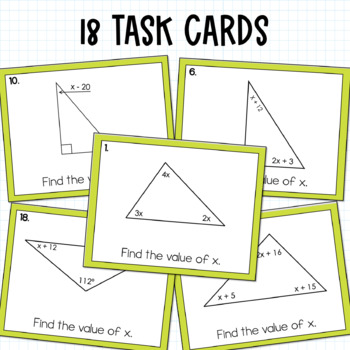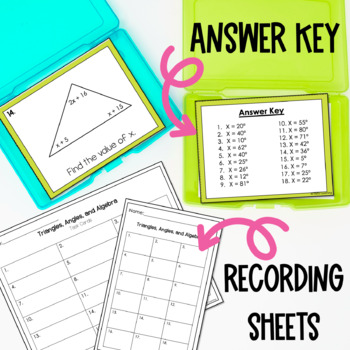# Triangles, Angles and Algebra Task Cards6th - 8th, Homeschool
Subjects
Standards
Resource Type
Formats Included
• PDF
Pages
12 pages

### Description

This set of 18 task cards give students practice finding unknown values by using their knowledge of:

triangles

the sum of angles in a triangle = 180 degrees

and

algebraic equations- writing and solving

combining like terms

each task card has a different triangle, and has each angle labeled with a variable expression or the right triangle notation. Students will need to write an equation using the variables for each angle and solve the equation to find the value of "x".

Students will get practice writing algebraic equations, combining like terms, solving equations and checking solutions for validity.

Two different student response sheets are included- one to respond with the value of "x" and the other to show the value of x and the equation they wrote to solve the problem.

For even more practice with angles and algebra, you may like:

Angle Measures and Algebra

Total Pages
12 pages
Included
Teaching Duration
Lifelong tool
Report this Resource to TpT
Reported resources will be reviewed by our team. Report this resource to let us know if this resource violates TpT’s content guidelines.

### Standards

to see state-specific standards (only available in the US).
Use facts about supplementary, complementary, vertical, and adjacent angles in a multi-step problem to write and solve simple equations for an unknown angle in a figure.